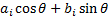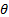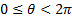/

### Extreme Events – Specimen Question A.4.2(b) – Answer/Hints

Q. Given a linear combination of Index A and B what is the maximum possible kurtosis of a linear combination of A and B?

The linear combination of the formwith the highest kurtosis can be found by finding the() which maximises the kurtosis of this combination. As kurtosis is scale invariant, this will also provide the maximum possible kurtosis. As A has a much higher kurtosis than B (4.62 vs -0.84) we expect that the resulting linear combination will be more biased towards A than was the one in (a) that maximised standard deviation and hence characterised the first principal component.

As in (a) we can find the solution using Microsoft Excel, e.g. using the Solver Add-in. It has= -0.088, i.e., as expected, close to A. The kurtosis of the resulting combination is 4.71.